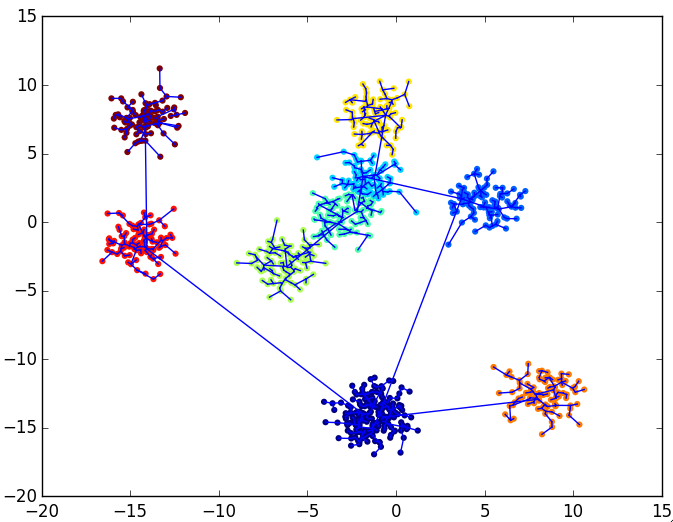7

1

4

1

# QuickShiftClustering

QuickShift  is a fast method for hierarchical clustering, which first constructs the clustering tree, and subsequently allows to quickly cut links in the tree which exceed a specified length. This second step can be performed for different link-lengths without having to re-run the clustering itself. Care has been taken to provide a high-performance implementation.

#### Functions

``````a = quickshift(data)
a = quickshift(data, sigma)
# cluster ndim x nsamplex matrix data.
# sigma: Gaussian kernel width, see paper

labels = quickshiftlabels(a::QuickShift)
# return cluster labels for data points.

quickshiftplot(a, data, labels)
# plot data points and hierarchical links
# needs PyPlot installed, only for 2D
``````

#### Performance

data 2 x N Runtime quickshift Runtime quickshiftlabels
1000 0.06 sec 0.0002 sec
10000 0.27 sec 0.004 sec
100000 9.67 sec 0.04 sec

For larger numbers of data points, you might want to use KShiftsClustering.jl to cluster the `N` data points to e.g. 10.000 cluster centers, and then perform QuickShift on those.

Comparison with `kmedoids` for 20.000 points:

``````using Clustering, QuickShiftClustering, FunctionalDataUtils

data = rand(2,20000)
@time a = kmedoids(1-exp(-distance(data,data)*10),10)
#  =>  elapsed time: 56.666481916 seconds (41126243444 bytes allocated, 15.31% gc time)

@time labels = quickshiftlabels(quickshift(data))
#  =>  elapsed time: 1.187448525 seconds (277816624 bytes allocated, 28.79% gc time)
``````

#### Example

``````using FunctionalData
data = @p map unstack(1:10) (x->10*randn(2,1).+randn(2,100)) | flatten

using QuickShiftClustering
a = quickshift(data)
labels = quickshiftlabels(a)

quickshiftplot(a, data, labels)
``````03/11/2015

4 months ago

1 commits